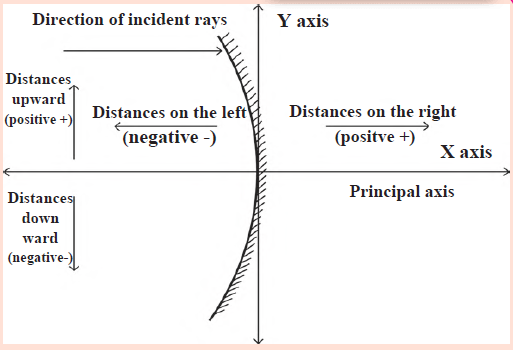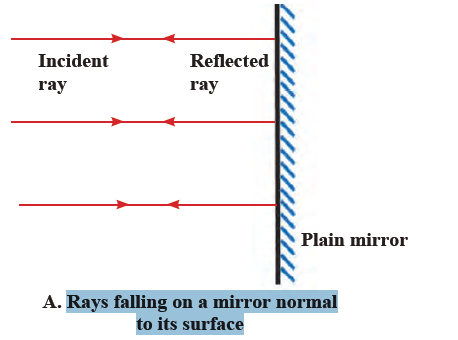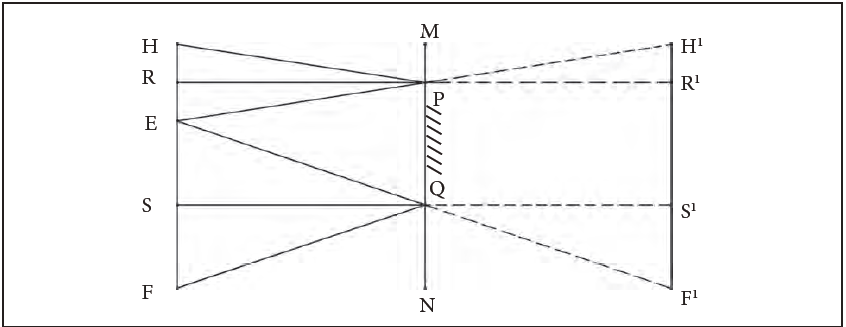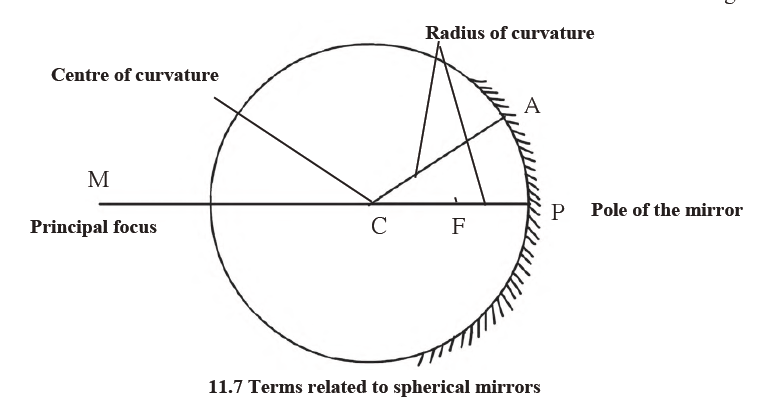# MSBSHSE Class 9 Science Chapter 11 Reflection of Light Solutions

MSBSHSE Class 9 Science Chapter 11 Reflection of Light Solutions is used by students to prepare for the exams. These solutions are offered here with detailed answers and proper step by step explanations. This will help the students to comprehend the basic concepts of this chapter thoroughly.

An important concept of Science, Reflection of Light is discussed at length in this Chapter and the solutions include questions from the chapter. Main topics include Mirrors and types of mirrors, Spherical mirrors and images formed by them, Magnification due to spherical mirrors and so on. Students can easily learn the subject thoroughly by revising these MSBSHSE Class 9 Solutions of Science Chapter 11 Reflection of Light. These solutions created after proper research will help students to gain a thorough conceptual understanding. The content is well structured and clear making it easier for the students to master the subject. These solutions explain the topics from the chapter of the textbook, as per the latest MSBSHSE Syllabus for Class 9. Practising these solutions to these questions listed here are designed to help students ace the exams.

## Maharashtra Board Class 9 Science Chapter 11- BYJU’S Important Questions & Answers

1.Explain the difference between a plane mirror, a concave mirror and a convex mirror with respect to the type and size of the images produced.

Answer: Plane mirror forms, virtual and erect images of the same size as the object. In the meantime, the images formed by the concave mirror vary with the position of the object with respect to the mirror. The image behind the concave mirror of the object positioned between the pole and the focus is an erect and virtual image that is magnified, while the image at infinity of the object at the focus is a very large inverted and real image. Also, the image beyond the centre of curvature for the object placed between focus and centre of curvature of mirror is magnified, inverted and real. Now, if the image and the object are the centre of the curvature, the real and inverted image will be the same size as the object. For images formed between the centre of curvature and focus for objects placed beyond the centre of curvature, the real and inverted images are diminished in size. Finally, the image formed at focus for an object placed at a very large (infinite) distance, the real and inverted image is of the size of a point. Alternately, the nature of the image formed by a convex mirror does not depend on the distance of the object from the mirror. These images are always virtual, smaller than the object and situated behind the mirror.

2. Describe the positions of the source of light with respect to a concave mirror in 1.Torch light

2. Projector lamp

3. Floodlight

Answer: Given here are the positions of the source of light with respect to a concave mirror:

1)Torch light- The source of light is positioned at the focus of the mirror. Thus, a parallel beam of light is obtained.

2) Projector lamp- Here, the source of light is located at the centre of curvature of the concave mirror.

3) Flood lights- Place the source of light a little beyond the centre of curvature of the mirror, thus giving off a bright beam of light.

3. Why are concave mirrors used in solar devices?

Answer: Concave mirrors are also known as converging mirrors as the sun rays reflected by a concave mirror come together in the focal plane. This feature or property of the concave mirror is used in solar devices. The parallel rays from the sun that are falling at a particular point produce a large amount of heat, which is used for proper functioning of the device.

4. Why are the mirrors fitted on the outside of cars convex?

Answer: Convex mirrors have the ability to form virtual and diminished images of the object, thus covering a wider field view. Hence, convex mirrors are equipped on the outside of cars so that the drivers can get a larger view of the road.The rear view mirrors in cars, which are curved mirrors enable drivers to see the vehicles coming from behind.

5. Why does obtaining the image of the sun on a paper with the help of a concave mirror burn the paper?

Answer: The parallel rays from the sun are converged to a single point on the focal plane of a mirror when these rays fall on the concave mirror. A large amount of heat is generated at this point. In the meantime, if a paper is positioned at that point to obtain the image of the sun, the paper will burn due to the huge amount of heat concentrated at this point.

6. If a spherical mirror breaks, what types of mirrors are the individual pieces?

Answer: Spherical mirrors are of two kinds- concave and convex mirrors. However, spherical mirrors do not change nature if broken. Hence, a concave mirror if broken will be two concave pieces, while the convex mirror will be two convex pieces.

7. What sign conventions are used for reflection from a spherical mirror?

Answer: Sign conventions as represented in the image below:As per the Cartesian sign convention, the pole of the mirror is considered as the origin and the principal axis is taken as the X- axis of the frame of reference.

Given below are the sign conventions:

1. The object is always kept on the left of the mirror. Measure all distances parallel to the principal axis from the pole of the mirror.

2. All distances measured towards the right of the pole are positive, while those measured towards the left are known to be negative.

3. Distances measured vertically upwards from the principal axis are taken to be positive.

4. Distances measured vertically downwards from the principal axis are considered negative.

5. The focal length of a concave mirror is negative, while that of a convex mirror is positive.

8. An object of height 7 cm is kept at a distance of 25 cm in front of a concave mirror. The focal length of the mirror is 15 cm. At what distance from the mirror should a screen be kept so as to get a clear image? What will be the size and nature of the image?

Answer: Following are the provided details,

h= 7cm

u = -25cm and

f=-15cm

Now, the distance of the screen from the mirror so as to obtain a clear image is taken as “v”.

Apply the mirror formula and get,

1/f = 1/v + 1/u

Therefore, 1/v = (1/-15) – (1/-25)

1/v = -2/75

Now, v = -37.5cm.

Screen should be at a distance of 37.5cm from the mirror.

After using the magnification formula, you get h1 / h = – (v/ u)

That is h1 = – (-37.5 / -25) × 7 = – 10.5 cm.

Thus, the size of the image is 10.5 cm and the negative sign is to indicate that the image is inverted and real.

9. A convex mirror has a focal length of 18 cm. The image of an object kept in front of the mirror is half the height of the object. What is the distance of the object from the mirror?

Answer: If f= 19cm and given that, h1 = h /2

h1 / h = ½ , then u = ?

Apply the magnification formula, h1 / h = – (v/ u)

v= -u × h1 / h = – u/2

To, this use the mirror formula, 1/f = 1/v + 1/u

That is 1/ 18 = – 2/ u + 1/ u

u=-18 cm.

So, the distance of the object from the mirror is 18cm.

10. What is light?

Answer: The electromagnetic radiation that causes the sensation of vision is known as Light.

11. What are the different types of reflection?

Answer: Light from the various surfaces reflects differently. For example, the reflection from smooth, flat surfaces is regular reflection, whereas rough surfaces reflect light irregularly.

12. What is a mirror, in scientific language? Types of mirrors used in daily life.

Answer: The mirror, in scientific language, is a polished surface that reflects light and produces clear images. It is a reflecting surface. Mirrors used in daily life are of two kinds- plane mirrors and spherical mirrors.

13. How is a plane mirror manufactured?

Answer: Used in several activities of daily life, the plane mirror is created by coating a thin, reflecting film of aluminium or silver at the back surface of a flat and smooth glass piece. Further, in order to protect this reflecting film and also to make that side opaque, another coat of a substance like lead oxide is done over it.

14. Illustrate the rays falling on a mirror normal to its surface with an image.15. When you place two plane mirrors at an angle of 90 degrees to each other and keep a small object between them, where will the image be formed?

Answer: If two plane mirrors are placed at a 90 degree angle to each other and a small object is positioned between them, then the image will be formed in both mirrors.

16. How is the number of images obtained relative to the angle of the mirrors placed?

Answer: If n is the number of images and A is the angle between the mirrors placed, then n = (360°/ A.) – 1.

17. As per the figure given, in order to see the full image of a person standing in front of a mirror, the minimum height of the mirror must be half the height of the person. Justify with proof.Answer: According to the image, H, E and F indicate the point at the top of the head, the eyes and a point at the feet of a person, respectively. Meanwhile, R and S are midpoints of HE and EF respectively. The mirror PQ is also at a height of NQ from the ground and is perpendicular to it. PQ is the minimum height of the mirror in order to obtain the full image of the person.

For this, RP and QS must be perpendicular to the mirror. Now, the minimum height of the mirror is PQ = RS = RE + ES = HE/ 2 + EF / 2 = HF/ 2 = half of the person’s height.

18. What is a Concave or Convex Mirror?

Answers: For a Concave mirror, the inner surface of the spherical mirror is the reflecting surface. Alternatively, for the Convex mirror, the outer surface of the spherical mirror is the reflecting surface.

19. Explain the terms related to spherical mirrors with the help of images.The pole of the Mirror denoted by P in the image is the centre of the mirror surface. Centre of the curvature, shown as C is the centre of the sphere of which the mirror is a part. Meanwhile, the length CP or CA indicated in the figure is the radius of curvature of the mirror or radius of the sphere. Another related term is the principal axis ‘PM’, which is a straight line passing through the pole and the centre of curvature of the mirror. The principal focus varies for Concave and Convex mirrors. The Incident rays that are parallel to the principal axis of a concave mirror, after it is reflected from the mirror, join at a particular point (F) called the principal focus in front of the mirror on the principal axis for the concave mirror. Similarly, in the case of a convex mirror, incident rays parallel to the principal axis, after reflection, appear to come from a particular point known as the principal focus behind the mirror lying along the principal axis for convex mirror. Finally, the focal length indicating the distance (f) between the pole and the principal focus of the mirror is half of the radius of curvature of the mirror.

20. Which are the rules used to draw a ray diagram? State them.

Answer: We observe certain rules based on the laws of reflection of light, while drawing a ray diagram. They are given here:

• Rule 1– If an incident ray is parallel to the principal axis, then the reflected ray passes through the principal focus.
• Rule 2– If an incident ray passes through the principal focus of the mirror, the reflected ray is parallel to the principal axis.
• Rule 3-If an incident ray passes through the centre of curvature of the mirror, the reflected ray traces the same path back.

21. Why are Concave mirrors called focussing mirrors?

Answer: A Concave mirror is also called a focusing mirror because parallel rays get focused after reflection in this mirror. The size of the image formed by these mirrors can be larger or smaller than the objects, on the basis of the distance of the object from the mirror.

22. Why are Convex mirrors called dispersing mirrors?

Answer: A convex mirror is also known as a dispersing mirror because parallel incident rays get dispersed after reflection in this mirror. Also, the size of the images generated by these mirrors are always smaller than the size of the objects.

23. What is the mirror formula? How is it expressed?

Answer: Mirror formula is the relationship between the object distance, image distance and the focal length (f). Now, given that the object distance (u) is the distance of the object from the pole and the image distance (v) is the distance of the image from the pole, then the mirror formula can be expressed as:

1/v + 1/u = 1/f

This is the valid formula for all spherical mirrors, for all positions of objects, under all circumstances.

24. How is magnification due to spherical mirrors expressed?

Answer: The magnification due to a spherical mirror is given by the ratio of the height of the image (h2) to the height of the object (h1). That is Magnification (M) = Height of the image / Height of the object = h2 / h1. From this we can conclude that M = – (v/u).

25. What is a real image and a virtual image?

Answer: Real image is the image in front of the mirror that can be obtained on a screen, whereas, the image that is behind the mirror and cannot be obtained on a screen is the virtual image.

26. Rajashree wants to get an inverted image of height 5 cm of an object kept at a distance of 30 cm from a concave mirror. The focal length of the mirror is 10 cm. At what distance from the mirror should she place the screen ? What will be the type of the image, and what is the height of the object?

Answer: Here it says that the focal length = f = -10cm, while the object distance(u) = – 30cm and height of the image (h2) =- 5 cm. Hence, we need to find image distance(v)= ? and height of the object(h1) = ?

Now, to get the image distance or v, you can apply the mirror formula here. That is,

1/v + 1/u = 1/f

1/v= (1/f) – (1/u)

1/ v = (1/ -10) – (1/ -30)

Hence, 1 / v = (- 3 + 1) / 30 = -2 / 30

1/ v = – 1 /15cm

v=-15cm

From this we can deduce that Rajashree has to keep the screen 15 cm to the left of the mirror.

Then to calculate the height of the object, you can use the magnification expression.

That is, M = h2 / h1= – (v/u)

Hence, h1 = – (uh2 / v)= – (-30) (-5) / -15

Therefore, h1 = ( -2) (-5) = 10 cm

So, the height of the object is 10cm. Thus, the image will be real and diminished.

Other than these MSBSHSE Class 9 Solutions, the students can access the textbooks, syllabus and sample papers to prepare for the exams. Stay tuned and get more information about the board exams from BYJU’S.